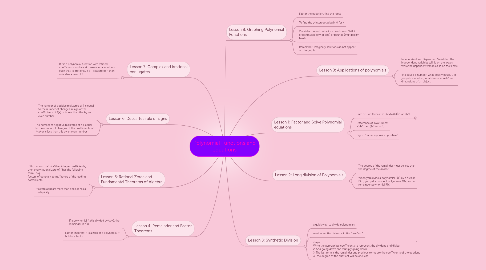# Polynomial Functions and equationsKailey Flaherty
Get Started. It's FreePolynomial Functions and equations## 5. Lesson 1: Factor and Solve Polynomial equations

### 5.1. Sum of Two Cubes: a³+b³=(a+b)(a²-ab+b²)

5.1.1. Difference of Two Cubes: a³-b³=(a+b)(a²+ab+b²)

5.1.2. Difference of Two Cubes: a³-b³=(a+b)(a²+ab+b²)

5.1.3. Difference of Two Cubes: a³-b³=(a+b)(a²+ab+b²)